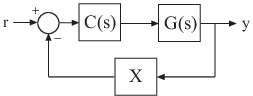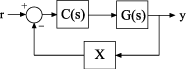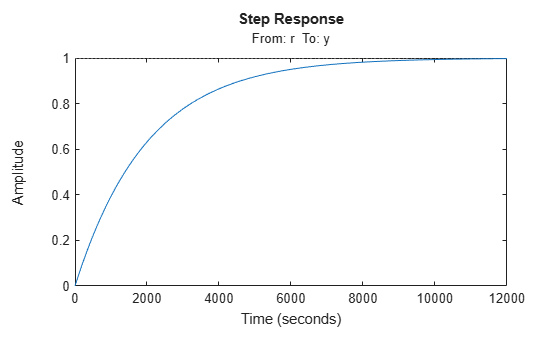Documentation

# AnalysisPoint

Points of interest for linear analysis

## Syntax

```AP = AnalysisPoint(name) AP = AnalysisPoint(name,N) ```

## Description

`AnalysisPoint` is a Control Design Block for marking a location in a control system model as a point of interest for linear analysis and controller tuning. You can combine an `AnalysisPoint` block with numeric LTI models, tunable LTI models, and other Control Design Blocks to build tunable models of control systems. `AnalysisPoint` locations are available for analysis with commands such as `getIOTransfer` or `getLoopTransfer`. Such locations are also available for specifying design goals for control system tuning.

For example, consider the following control system.Suppose that you are interested in the effects of disturbance injected at u in this control system. Inserting an `AnalysisPoint` block at the location u associates an implied input, implied output, and the option to open the loop at that location, as in the following diagram.Suppose that `T` is a model of the control system including the `AnalysisPoint` block, `AP_u`. In this case, the command `getIOTransfer(T,'AP_u','y')` returns a model of the closed-loop transfer function from u to y. Likewise, the command `getLoopTransfer(T,'AP_u',-1)` returns a model of the negative-feedback open-loop response, CG, measured at the location u.

`AnalysisPoint` blocks are also useful when tuning a control system using tuning commands such as `systune`. You can use an `AnalysisPoint` block to mark a loop-opening location for open-loop tuning requirements such as `TuningGoal.LoopShape` or `TuningGoal.Margins`. You can also use an `AnalysisPoint` block to mark the specified input or output for tuning requirements such as `TuningGoal.Gain`. For example, `Req = TuningGoal.Margins('AP_u',5,40)` constrains the gain and phase margins at the location u.

You can create `AnalysisPoint` blocks explicitly using the `AnalysisPoint` command and connect them with other block diagram components using model interconnection commands. For example, the following code creates a model of the system illustrated above. (See Construction and Examples below for more information.)

```G = tf(1,[1 2]); C = tunablePID('C','pi'); AP_u = AnalysisPoint('u'); T = feedback(G*AP_u*C,1); % closed loop r->y```

You can also create analysis points implicitly, using the `connect` command. The following syntax creates a dynamic system model with analysis points, by interconnecting multiple models `sys1,sys2,...,sysN`:

`sys = connect(sys1,sys2,...,sysN,inputs,outputs,APs);`

`APs` lists the signal locations at which to insert analysis points. The software automatically creates and inserts an `AnalysisPoint` block with channels corresponding to these locations. See `connect` for more information.

## Construction

`AP = AnalysisPoint(name)` creates a single-channel analysis point. Insert `AP` anywhere in the generalized model of your control system to mark a point of interest for linear analysis or controller tuning. `name` specifies the block name.

`AP = AnalysisPoint(name,N)` creates a multi-channel analysis point with `N` channels. Use this block to mark a vector-valued signal as a point of interest or to bundle together several points of interest.

### Input Arguments

 `name` Analysis point name, specified as a character vector such as `'AP'`. This input argument sets the value of the `Name` property of the `AnalysisPoint` block. (See Properties.) When you build a control system model using the block, the `Name` property is what appears in the `Blocks` list of the resulting `genss` model. `N` Number of channels for a multichannel analysis point, specified as a scalar integer.

## Properties

 `Location` Names of channels in the `AnalysisPoint` blocks, specified as a character vector or a cell array of character vectors. By default, the analysis-point channels are named after the `name` argument. For example, if you have a single-channel analysis point, `AP`, that has name `'AP'`, then ```AP.Location = 'AP'``` by default. If you have a multi-channel analysis point, then `AP.Location = {'AP(1)','AP(2)',...}` by default. Set `AP.Location` to a different value if you want to customize the channel names. `Open` Loop-opening state, specified as a logical value or vector of logical values. This property tracks whether the loop is open or closed at the analysis point. For example, consider the feedback loop of the following illustration.You can model this feedback loop as follows. ```G = tf(1,[1 2]); C = tunablePID('C','pi'); X = AnalysisPoint('X'); T = feedback(G*C,X);``` You can get the transfer function from r to y with the feedback loop open at X as follows. ` Try = getIOTransfer(T,'r','y','X');` In the resulting generalized state-space (`genss`) model, the `AnalysisPoint` block `'X'` is marked open. In other words, `Try.Blocks.X.Open = 1`. For a multi-channel analysis point, then `Open` is a logical vector with as many entries as the analysis point has channels. Default: 0 for all channels `Ts` Sample time. For `AnalysisPoint` blocks, the value of this property is automatically set to the sample time of other blocks and models you connect it with. Default: `0` (continuous time) `TimeUnit` Units for the time variable, the sample time `Ts`, and any time delays in the model, specified as one of the following values:`'nanoseconds'``'microseconds'``'milliseconds'``'seconds'` `'minutes'``'hours'``'days'``'weeks'``'months'``'years'` Changing this property has no effect on other properties, and therefore changes the overall system behavior. Use `chgTimeUnit` to convert between time units without modifying system behavior. Default: `'seconds'` `InputName` Input channel names, specified as one of the following: Character vector — For single-input models, for example, `'controls'`.Cell array of character vectors — For multi-input models. Alternatively, use automatic vector expansion to assign input names for multi-input models. For example, if `sys` is a two-input model, enter: `sys.InputName = 'controls';` The input names automatically expand to `{'controls(1)';'controls(2)'}`. You can use the shorthand notation `u` to refer to the `InputName` property. For example, `sys.u` is equivalent to `sys.InputName`. Input channel names have several uses, including: Identifying channels on model display and plotsExtracting subsystems of MIMO systemsSpecifying connection points when interconnecting models Default: `''` for all input channels `InputUnit` Input channel units, specified as one of the following: Character vector — For single-input models, for example, `'seconds'`.Cell array of character vectors — For multi-input models. Use `InputUnit` to keep track of input signal units. `InputUnit` has no effect on system behavior. Default: `''` for all input channels `InputGroup` Input channel groups. The `InputGroup` property lets you assign the input channels of MIMO systems into groups and refer to each group by name. Specify input groups as a structure. In this structure, field names are the group names, and field values are the input channels belonging to each group. For example: ```sys.InputGroup.controls = [1 2]; sys.InputGroup.noise = [3 5];``` creates input groups named `controls` and `noise` that include input channels 1, 2 and 3, 5, respectively. You can then extract the subsystem from the `controls` inputs to all outputs using: `sys(:,'controls')` Default: Struct with no fields `OutputName` Output channel names, specified as one of the following: Character vector — For single-output models. For example, `'measurements'`.Cell array of character vectors — For multi-output models. Alternatively, use automatic vector expansion to assign output names for multi-output models. For example, if `sys` is a two-output model, enter: `sys.OutputName = 'measurements';` The output names automatically expand to `{'measurements(1)';'measurements(2)'}`. You can use the shorthand notation `y` to refer to the `OutputName` property. For example, `sys.y` is equivalent to `sys.OutputName`. Output channel names have several uses, including: Identifying channels on model display and plotsExtracting subsystems of MIMO systemsSpecifying connection points when interconnecting models Default: `''` for all output channels `OutputUnit` Output channel units, specified as one of the following: Character vector — For single-output models. For example, `'seconds'`.Cell array of character vectors — For multi-output models. Use `OutputUnit` to keep track of output signal units. `OutputUnit` has no effect on system behavior. Default: `''` for all output channels `OutputGroup` Output channel groups. The `OutputGroup` property lets you assign the output channels of MIMO systems into groups and refer to each group by name. Specify output groups as a structure. In this structure, field names are the group names, and field values are the output channels belonging to each group. For example: ```sys.OutputGroup.temperature = ; sys.InputGroup.measurement = [3 5];``` creates output groups named `temperature` and `measurement` that include output channels 1, and 3, 5, respectively. You can then extract the subsystem from all inputs to the `measurement` outputs using: `sys('measurement',:)` Default: Struct with no fields `Name` System name, specified as a character vector. For example, `'system_1'`. Default: `''` `Notes` Any text that you want to associate with the system, stored as a string or a cell array of character vectors. The property stores whichever data type you provide. For instance, if `sys1` and `sys2` are dynamic system models, you can set their `Notes` properties as follows: ```sys1.Notes = "sys1 has a string."; sys2.Notes = 'sys2 has a character vector.'; sys1.Notes sys2.Notes``` ```ans = "sys1 has a string." ans = 'sys2 has a character vector.' ``` Default: `[0×1 string]` `UserData` Any type of data you want to associate with system, specified as any MATLAB® data type. Default: `[]`

## Examples

collapse all

Create a model of the following feedback loop with an analysis point in the feedback path.For this example, the plant model is $G=1/\left(s+2\right)$. C is a tunable PI controller, and X is the analysis point.

```G = tf(1,[1 2]); C = tunablePID('C','pi'); X = AnalysisPoint('X'); T = feedback(G*C,X); T.InputName = 'r'; T.OutputName = 'y';```

`T` is a tunable `genss` model. `T.Blocks` contains the Control Design Blocks of the model, which are the controller, `C`, and the analysis point, `X`.

`T.Blocks`
```ans = struct with fields: C: [1x1 tunablePID] X: [1x1 AnalysisPoint] ```

Examine the step response of `T`.

`stepplot(T)`The presence of the `AnalysisPoint` block does not change the dynamics of the model.

You can use the analysis point for linear analysis of the system. For instance, extract the system response at `'y'` to a disturbance injected at the analysis point.

`Txy = getIOTransfer(T,'X','y');`

The `AnalysisPoint` block also allows you to temporarily open the feedback loop at that point. For example, compute the open-loop response from `'r'` to `'y'`.

`Try_open = getIOTransfer(T,'r','y','X');`

Specifying the analysis point name as the last argument to getIOTransfer extracts the response with the loop open at that point. Examine the step response of `Try_open` to verify that it is the open-loop response.

`stepplot(Try_open);`Create a model of the following block diagram from r to y. Insert an analysis point at an internal location, u.Create `C` and `G`, and name the inputs and outputs.

```C = pid(2,1); C.InputName = 'e'; C.OutputName = 'u'; G = zpk([],[-1,-1],1); G.InputName = 'u'; G.OutputName = 'y';```

Create the summing junction.

`Sum = sumblk('e = r - y');`

Combine `C`, `G`, and the summing junction to create the aggregate model, with an analysis point at u.

`T = connect(G,C,Sum,'r','y','u')`
```T = Generalized continuous-time state-space model with 1 outputs, 1 inputs, 3 states, and the following blocks: AnalysisPoints_: Analysis point, 1 channels, 1 occurrences. Type "ss(T)" to see the current value, "get(T)" to see all properties, and "T.Blocks" to interact with the blocks. ```

The resulting `T` is a `genss` model. The `connect` command creates the `AnalysisPoint` block, `AnalysisPoints_`, and inserts it into `T`. To see the name of the analysis point channel in `AnalysisPoints_`, use `getPoints`.

`getPoints(T)`
```ans = 1x1 cell array {'u'} ```

The analysis point channel is named `'u'`. You can use this analysis point to extract system responses. For example, the following commands extract the open-loop transfer at u and the closed-loop response at y to a disturbance injected at u.

```L = getLoopTransfer(T,'u',-1); Tuy = getIOTransfer(T,'u','y');```

`T` is equivalent to the following block diagram, where AP_u designates the `AnalysisPoint` block `AnalysisPoints_` with channel name u.Create a block for marking two analysis points in a MIMO model.

In the control system of the following illustration, consider each signal a vector-valued signal of size 2. In other words, the signal $r$ represents `{r(1),r(2)}`, $y$ represents `{y(1),y(2)}`, and so on.The feedback signal is therefore also a vector-valued signal of size 2. Create a block for marking the two analysis points in the feedback path.

`AP = AnalysisPoint('X',2)`
```AP = Multi-channel analysis point at locations: X(1) X(2) Type "ss(AP)" to see the current value and "get(AP)" to see all properties. ```

The `AnalysisPoint` block is stored as a variable in the MATLAB® workspace called `AP`. In addition, the `Name` property of the block is set to `X`. When you interconnect the block with numeric LTI models or other Control Design Blocks, this analysis-point block is identified in the `Blocks` property of the resulting `genss` model as `X`. The block name `X` is automatically expanded to generate the channel names `X(1)` and `X(2)`.

It is sometimes convenient to change the channel names to match the names of the signals they correspond to in a block diagram of your model. For example, suppose the points of interest you want to mark in your model are signals named `L` and `V`. Change the `Location` property of `AP` to make the names match those signals.

`AP.Location = {'L';'V'}`
```AP = Multi-channel analysis point at locations: L V Type "ss(AP)" to see the current value and "get(AP)" to see all properties. ```

Although the channel names have changed, the block name remains `X`.

`AP.Name`
```ans = 'X' ```

Therefore, the `Blocks` property of a `genss` model you build with this block still identifies the block as `X`. Use `getPoints` to find the channel names of available analysis points in a `genss` model.